# Fraction calculator

The calculator performs basic and advanced operations with fractions, expressions with fractions combined with integers, decimals, and mixed numbers. It also shows detailed step-by-step information about the fraction calculation procedure. Solve problems with two, three, or more fractions and numbers in one expression.

## Result:

### 9/12 - 5/12 = 1/3 ≅ 0.3333333

Spelled result in words is one third.

### How do you solve fractions step by step?

1. Subtract: 9/12 - 5/12 = 9 - 5/12 = 4/12 = 4 · 1/4 · 3 = 1/3
For adding, subtracting, and comparing fractions, it is suitable to adjust both fractions to a common (equal, identical) denominator. The common denominator you can calculate as the least common multiple of both denominators - LCM(12, 12) = 12. In practice, it is enough to find the common denominator (not necessarily the lowest) by multiplying the denominators: 12 × 12 = 144. In the following intermediate step, cancel by a common factor of 4 gives 1/3.
In other words - nine twelfths minus five twelfths = one third.

#### Rules for expressions with fractions:

Fractions - simply use a forward slash between the numerator and denominator, i.e., for five-hundredths, enter 5/100. If you are using mixed numbers, be sure to leave a single space between the whole and fraction part.
The slash separates the numerator (number above a fraction line) and denominator (number below).

Mixed numerals (mixed fractions or mixed numbers) write as integer separated by one space and fraction i.e., 1 2/3 (having the same sign). An example of a negative mixed fraction: -5 1/2.
Because slash is both signs for fraction line and division, we recommended use colon (:) as the operator of division fractions i.e., 1/2 : 3.

Decimals (decimal numbers) enter with a decimal point . and they are automatically converted to fractions - i.e. 1.45.

The colon : and slash / is the symbol of division. Can be used to divide mixed numbers 1 2/3 : 4 3/8 or can be used for write complex fractions i.e. 1/2 : 1/3.
An asterisk * or × is the symbol for multiplication.
Plus + is addition, minus sign - is subtraction and ()[] is mathematical parentheses.
The exponentiation/power symbol is ^ - for example: (7/8-4/5)^2 = (7/8-4/5)2

#### Examples:

subtracting fractions: 2/3 - 1/2
multiplying fractions: 7/8 * 3/9
dividing Fractions: 1/2 : 3/4
exponentiation of fraction: 3/5^3
fractional exponents: 16 ^ 1/2
adding fractions and mixed numbers: 8/5 + 6 2/7
dividing integer and fraction: 5 ÷ 1/2
complex fractions: 5/8 : 2 2/3
decimal to fraction: 0.625
Fraction to Decimal: 1/4
Fraction to Percent: 1/8 %
comparing fractions: 1/4 2/3
multiplying a fraction by a whole number: 6 * 3/4
square root of a fraction: sqrt(1/16)
reducing or simplifying the fraction (simplification) - dividing the numerator and denominator of a fraction by the same non-zero number - equivalent fraction: 4/22
expression with brackets: 1/3 * (1/2 - 3 3/8)
compound fraction: 3/4 of 5/7
fractions multiple: 2/3 of 3/5
divide to find the quotient: 3/5 ÷ 2/3

The calculator follows well-known rules for order of operations. The most common mnemonics for remembering this order of operations are:
PEMDAS - Parentheses, Exponents, Multiplication, Division, Addition, Subtraction.
BEDMAS - Brackets, Exponents, Division, Multiplication, Addition, Subtraction
BODMAS - Brackets, Of or Order, Division, Multiplication, Addition, Subtraction.
GEMDAS - Grouping Symbols - brackets (){}, Exponents, Multiplication, Division, Addition, Subtraction.
Be careful, always do multiplication and division before addition and subtraction. Some operators (+ and -) and (* and /) has the same priority and then must evaluate from left to right.

## Fractions in word problems:

• The recipeThe recipe they are following requires 7/8 cups of milk, Tom already put 3/8 cups of milk. How much milk should Lea add to follow the recipe?
• Hotel 4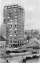A 360 room hotel has 1/3 of its rooms occupied at present. How many rooms are empty?
• 5 2/55 2/5 hours a week  mathematics,  3 3/4 hours a week   Natural sciences, 4 3/8 hours a week  Technology . how many hours does  he spend on social sciences if he spend 17 1/2 hours a week for the four subject?
• Half of halvesHalf of the square we cut off, then half of the rest, etc. Five cuts we made in this way. What part of the content of the original square is the content of the cut part?About 6/9 of the sixth- grade pupils will be going to the parents' seminar. If 1/6 of the participants are girls, what part of the portion of sixth graders are boys?
• PoundsThree pounds subtract 1/3 of a pound.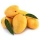A basket contains three types of fruits weighing 87/4 kg in all. If 23/4 kilograms of these are oranges, 48/7 kg are mangoes, and the rest are apples. What is the weight of the apples in the basket?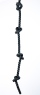From a rope that is 11 m long, two pieces of lengths 13/5 m and 33/10 m are cut off. What is the length of the remaining rope?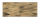Tina and Bill share a 12-ounce bucket of clay. By the end of the week, Tina has used 1/6 of the bucket, and Bill has used 2/3 of the bucket of clay. How many ounces are left in the bucket?We cut out four squares of 300 mm side from a square sheet metal plate with a side of 0,7 m. Express the fraction and the percentage of waste from the square metal sheet.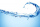Benhur boiled 1 1/4 liters of water in a kettle. After 10 1/2 minutes he measured the water again. It had 3/4 liters left in the kettle. What is the amount of water that evaporates every minutes?What is the product of two fourths  and the sum of three halves and four?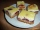There is 3/4 of a cake on a plate in Maria's kitchen.  Silvia sees the cake and eats 1/5 of the cake.  Then Franca takes 1/3 of what was there and shares half of her portion with Antonella.  What fraction of the cake is left?